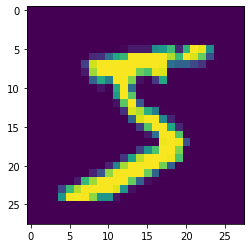# Tensorflow Hello World

5 minute read   Share on :

Tensorflow is made up of two words tensor and flow , where tensor means multidimensional array and flow means graph of operations. It is developed by google brains team. It is released under Apache 2.0 license. It is a package in python and concurrently spreading in other languages such as R , Julia etc. Tensorflow have very smooth learning curve and it is easy for newcomers to grasp vast machine learning easily.

To get started we have download python anaconda version , that will automatically install jupyter notebook and then install Tensorflow to so this read our article here

You have to type Shift+Enter to run a cell in jupyter notebook

We will use MNIST dataset , which is developed by Yann LeCun, Courant Institute, NYU Corinna Cortes, Google Labs, New York and Christopher J.C. Burges, Microsoft Research, Redmond , in the dataset there are 60,000 images of handwritten digits and labeled them for training, and 10,000 images for testing. MNIST dataset is already divided in test and train set so we do not have to take care of that .

First of all we have to import tensorflow , with an alias tf to use tensorflow

import tensorflow as tf


Now we will import dataset and store it

• x_train the trainig images
• y_train label of the training images
• x_test testing images
• y_test label of the testing images
mnist = tf.keras.datasets.mnist
(x_train, y_train), (x_test, y_test) = mnist.load_data()


Now let us take a look at first image of the handwritten digit

from matplotlib import pyplot
pyplot.imshow(x_train)
pyplot.show()Now we will divide x_train and x_test by 255, because our image is RGB so each pixel in our image can take any value between 0 to 255 , and neural networks works fine with range from 0 to 1 , so to normalize our dataset in 0 to 1 we divide both train and tes dataset by 255

x_train, x_test = x_train / 255.0, x_test / 255.0


Now we need to create a model we will use tf.keras.models.Sequential to create a model and we will use four layers in it from the module tf.keras.layers, layers are as follows

• tf.keras.layers.Flatten : it will flatten our data , our image is 28 $\times$ 28 pixels , it will flatten the image and convert it into 784 $\times$ 1 , it will take argument input_shape which will be a tuple that define the shape of our input data

• tf.keras.layers.Dense : it is just a layer with units here 128, and a activation function here relu

• tf.keras.layers.Dropout : This layer drop input with a probability of rate(here 0.2) and multiply each non dropped input by $\frac{1}{1-rate}$
• tf.keras.layers.Dense : it is similar to the second layer
• tf.keras.layers.Softmax: it is used because output of the dense layer will be log-odds , softmax function maps logodds to probabilities
model = tf.keras.models.Sequential([
tf.keras.layers.Flatten(input_shape=(28, 28)),
tf.keras.layers.Dense(128, activation='relu'),
tf.keras.layers.Dropout(0.2),
tf.keras.layers.Dense(10),
tf.keras.layers.Softmax()
])


So for a input i.e 28 $\times$ 28 image , in the model, it gives us an array of 10 floating point number that will be output of the last dense layer.Let us get a output without training the model

predictions = model(x_train[:1]).numpy()
predictions

££ array([[0.09967916, 0.09987953, 0.09993076, 0.10024416, 0.10007039,
££       0.10004147, 0.10008495, 0.09998867, 0.10009976, 0.09998112]],
££       dtype=float32)


Now we can check our model using model.summary()

model.summary()

££  Model: "sequential_5"
££  _________________________________________________________________
££  Layer (type)                 Output Shape              Param #
££  =================================================================
££  flatten_7 (Flatten)          (None, 784)               0
££  _________________________________________________________________
££  dense_13 (Dense)             (None, 128)               100480
££  _________________________________________________________________
££  dropout_7 (Dropout)          (None, 128)               0
££  _________________________________________________________________
££  dense_14 (Dense)             (None, 10)                1290
££  _________________________________________________________________
££  softmax_1 (Softmax)          (None, 10)                0
££  =================================================================
££  Total params: 101,770
££  Trainable params: 101,770
££  Non-trainable params: 0
££  _________________________________________________________________


Now we will define a loss function , we should choose loss function such as if our model predict wrong label our loss will , tensorflow have a lots of inbuilt loss function , here we will use Sparse Categorical Cross entropy, for in detailed description check here

loss_fn = tf.keras.losses.SparseCategoricalCrossentropy()


Now our main target is to set trainable params such that we get minimum loss , and we are using accuracy to measure the performance it can be calculated by dividing true class prediction by total predictions , we will use adam as a optimizer

model.compile(optimizer='adam',
loss=loss_fn,
metrics=['accuracy'])


Now let us train our model

model.fit(x_train, y_train, epochs=5)

££  Epoch 1/5
££  1875/1875 [==============================] - 5s 3ms/step - loss: 0.1909 - accuracy: 0.9445
££  Epoch 2/5
££  1875/1875 [==============================] - 5s 3ms/step - loss: 0.1854 - accuracy: 0.9465
££  Epoch 3/5
££  1875/1875 [==============================] - 5s 3ms/step - loss: 0.1821 - accuracy: 0.9471
££  Epoch 4/5
££  1875/1875 [==============================] - 5s 3ms/step - loss: 0.1773 - accuracy: 0.9493
££  Epoch 5/5
££  1875/1875 [==============================] - 5s 3ms/step - loss: 0.1741 - accuracy: 0.9498


Now let us evaluate our model on test set

model.evaluate(x_test,  y_test, verbose=2)

££ 313/313 - 1s - loss: 0.1480 - accuracy: 0.9569
££ [0.14800186455249786, 0.9569000005722046]


As we can see we have approx ~95% accuracy

Here we have created a model , trained it , make predictions on it.

Happy Learning

Tags:

Categories:

Updated: